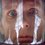# Playing with Integrals: Inequalities and Integrals 1

Integration, just as derivation, reveals a new approach to proving the inequalities. Let's take a detailed view on inequalities solved by or involving intgrals.

Theorem 1. If for all $$x\in[a,b]$$ the following inequality holds $f(x)\geq g(x),$ then for all $$x\in[a,b]$$ we have $\int^x_af(t)\,dt\geq\int^x_ag(t)\,dt.$

I won't present a formal proof, but rather just a simple image to ilustrate the idea.Now armed with this theorem let's solve few very basic problems.

Problem 1. Prove the following inequality $\ln(2\sin x)>\frac{1}{2}x(\pi-x)-\frac{5}{72}\pi^2,\forall x\in\left(\frac{\pi}{6},\frac{\pi}{2}\right)$

Solution. We know the very basic inequality $\tan x>x$ for $0< x <\dfrac{\pi}{2}$. Now if we substitute $x$ with $\dfrac{\pi}{2}-x$ we'll obtain $\cot x>\dfrac{\pi}{2}-x$. Now integrating our inequality we obtain $\int^x_{\frac{\pi}{6}}\cot t\,dt>\int^x_{\frac{\pi}{6}}\left(\dfrac{\pi}{2}-t\right)\,dt.$ Calculating we get $\ln(\sin x)-\ln\frac{1}{2}>\left(\frac{\pi x}{2}-\frac{x^2}{2}\right)-\left(\frac{\pi}{2}\cdot\frac{\pi}{6}-\frac{1}{2}\left(\frac{\pi^2}{6}\right)\right)\Leftrightarrow$ $\Leftrightarrow \ln(2\sin x)>\frac{1}{2}x(\pi-x)-\frac{5}{72}\pi^2.$

Problem 2. For $0< x <\dfrac{\pi}{2}$ prove the following inequality $\sin x \leq \frac{x(\pi-x)}{2}.$

Problem 3. For $b\geq 1$ prove the following inequality $ab\leq e^a+b(\ln b -1).$Note by Nicolae Sapoval
6 years, 7 months ago

This discussion board is a place to discuss our Daily Challenges and the math and science related to those challenges. Explanations are more than just a solution — they should explain the steps and thinking strategies that you used to obtain the solution. Comments should further the discussion of math and science.

When posting on Brilliant:

• Use the emojis to react to an explanation, whether you're congratulating a job well done , or just really confused .
• Ask specific questions about the challenge or the steps in somebody's explanation. Well-posed questions can add a lot to the discussion, but posting "I don't understand!" doesn't help anyone.
• Try to contribute something new to the discussion, whether it is an extension, generalization or other idea related to the challenge.

MarkdownAppears as
*italics* or _italics_ italics
**bold** or __bold__ bold
- bulleted- list
• bulleted
• list
1. numbered2. list
1. numbered
2. list
Note: you must add a full line of space before and after lists for them to show up correctly
paragraph 1paragraph 2

paragraph 1

paragraph 2

[example link](https://brilliant.org)example link
> This is a quote
This is a quote
    # I indented these lines
# 4 spaces, and now they show
# up as a code block.

print "hello world"
# I indented these lines
# 4 spaces, and now they show
# up as a code block.

print "hello world"
MathAppears as
Remember to wrap math in $$ ... $$ or $ ... $ to ensure proper formatting.
2 \times 3 $2 \times 3$
2^{34} $2^{34}$
a_{i-1} $a_{i-1}$
\frac{2}{3} $\frac{2}{3}$
\sqrt{2} $\sqrt{2}$
\sum_{i=1}^3 $\sum_{i=1}^3$
\sin \theta $\sin \theta$
\boxed{123} $\boxed{123}$

Sort by:

thanks! :)

- 6 years ago

Very nice problems keep it up nicolae

- 6 years, 3 months ago

Sir, Can You give Solution to Problem 3,,I am not able to do it. I have done With 2nd one

- 6 years, 4 months ago

I could only solve it by considering two cases. Case 1. If $a>x> \ln b \geq 0$ then in this interval $e^x \geq b \geq 1$. Thus $\displaystyle \int_{\ln b}^{a} e^x \mathrm{d}x \geq \displaystyle \int_{\ln b}^{a} b \mathrm{d}x$. On expanding we have $e^a-b \geq b(a-\ln b) \Rightarrow ab \leq e^a+ b(\ln b -1)$. $\space$ Case 2 If $\ln b \geq x \geq 0 >a$ then in this interval $b\geq e^x$. Thus $\displaystyle \int_{a}^{\ln b} b\mathrm{d}x \geq \displaystyle \int_{a}^{\ln b} e^x \mathrm{d}x$. Which on expanding gives us $b(\ln b -a) \geq b- e^a \Rightarrow ab \geq e^a + b(\ln b -1)$. PS: Let me know if you find a mistake or an easier way, all criticisms are welcomed.

- 6 years, 4 months ago

This is actually a really interesting topic that I have never heard of! Thanks for sharing!

- 6 years, 6 months ago